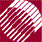ECE532 Biomedical Optics © 1998 Steven L. Jacques, Scott A. Prahl Oregon Graduate Institute

# Time-resolved Monte Carlo

## Initialize variables

Once the user has selected the variable settings, the program begins by initializing certain variables and zeroing the arrays.

• the incremental bin size, dr, is set to the total radial range under consideration divided by the number of bins in the data array.
• the speed of light in the medium, s, is calculated based on the tissue refractive index.
• the photon counter, i_photon, is zeroed.
• the random number generator is initialized with a seed number.
• the pathlengths, LT[it] = T[it]*c, are calculated.
• the maximum pathlength, Lmax, is specified.
• the data array, Csph[ir][it], is zeroed.
```/**** INITIALIZATIONS
*****/
dr = radial_size/NR;      /* cm,  incremental bin size */
c = LIGHTSPEED/nt;        /* lightspeed within medium */
i_photon = 0;             /* zero photon counter */
InitRandomGen;            /* initialize seed of random number generator */

for (it=0; it<NTpts; it++)
LT[it] = T[it]*c;     /* pathlengths LT[it] associated with T[it] */
Lmax  = LT[NTpts-1];      /* last total pathlength considered */

for (ir=0; ir<=NR; ir++)  /* clear data array */
for (it=0; it<NTpts; it++)
Csph[ir][it] = 0;
```

NextMonte Carlo